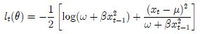# Differentiate log likelihood

#### Subhotosh Khan

##### Super Moderator
Staff member
Hi,
Can someone help me and explain step-by-step how to partial differentiate the following log-likelihood (see picture) wrt. mu (the sign that looks like u).
Also, how to differentiate the same equation wrt. omega (the sign that looks like w).

View attachment 15993

Thank youIf I were to do the problem, I'll first simplify (to my eye only) as follows:

$$\displaystyle l_t(\theta) \ = -\frac{1}{2} * \left [ log (B) + \frac{(x_t - \mu)^2}{B}\right ]$$ .... where B and xt are constants (for this instant)

Now differentiate above w.r.t. $$\displaystyle \mu$$